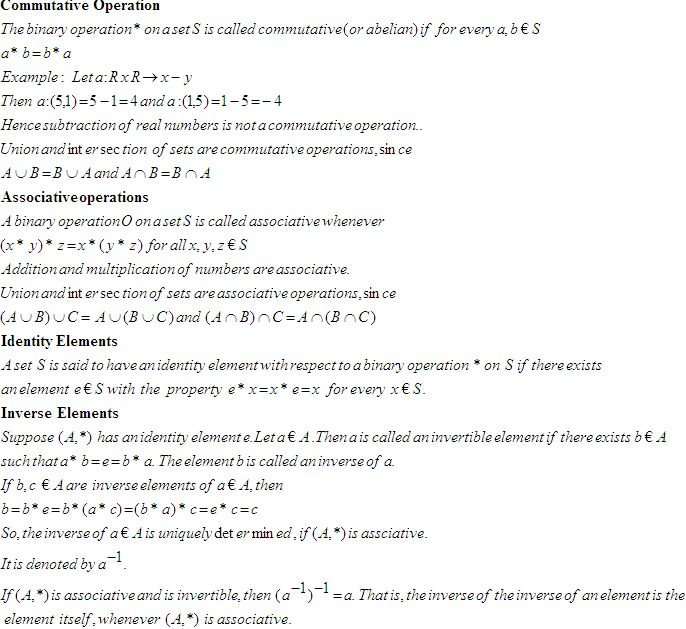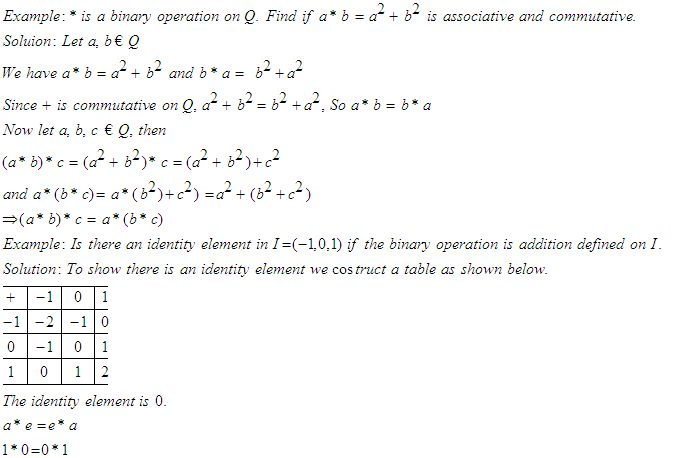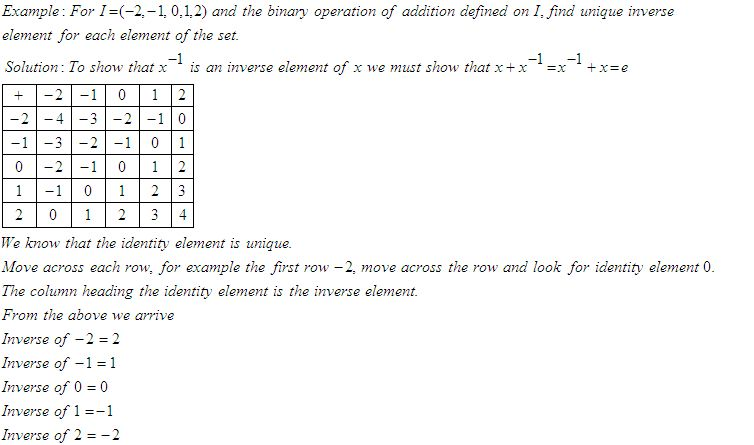Email us to get an instant 20% discount on highly effective K-12 Math & English kwizNET Programs!

#### Online Quiz (WorksheetABCD)

Questions Per Quiz = 2 4 6 8 10

### High School Mathematics - 25.2 Laws of Binary OperationsDirections: Answer the following.
 Q 1: For I = (-2,-1,0,1,2) and the binary operation of addition defined on I, ehat is the inverse of -2.210 Q 2: Let * be a binary operation defined on Q. Check if a*b = a2+b2, for a,b € Q is commutative.YesNo Q 3: Let a*b = l.c.m of (a,b), a, b € N, find 3*512815 Q 4: Find the identity element if a*b = a+b+ab , a, b € 1Answer: Q 5: Let A = N x N and let * be a binary operation on A defined by (a,b)*(c,d) = (ac, bd). Show that (A,*) is associative.Answer: Q 6: Let * be a binary operation defined on Q. Check if a*b = a-b, for a,b € Q is associative.Answer: Q 7: Let * be a binary operation defined on Q. Check if a*b = a-b, for a,b € Q is commutative.NoYes Q 8: Let a * b be a binary operation on N given by a * b = l.c.m (a,b), a, b, € N, find 2*4842 Question 9: This question is available to subscribers only! Question 10: This question is available to subscribers only!

#### Subscription to kwizNET Learning System offers the following benefits:

• Unrestricted access to grade appropriate lessons, quizzes, & printable worksheets
• Instant scoring of online quizzes
• Progress tracking and award certificates to keep your student motivated
• Unlimited practice with auto-generated 'WIZ MATH' quizzes